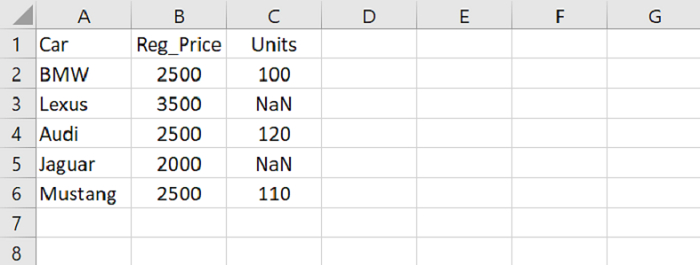# Python Pandas - Fill NaN values using an interpolation method

PythonServer Side ProgrammingProgramming

Use the interpolate() method to fill NaN values. Let’s say the following is our CSV file opened in Microsoft Excel with some NaN values −Load data from a CSV file into a Pandas DataFrame −

dataFrame = pd.read_csv("C:\Users\amit_\Desktop\SalesData.csv")

Fill NaN values with interpolate() −

dataFrame.interpolate()

## Example

Following is the code −

import pandas as pd

# Load data from a CSV file into a Pandas DataFrame
print("DataFrame...\n",dataFrame)

# fill NaN values with interpolate()
res = dataFrame.interpolate()
print("\nDataFrame after interpolation...\n",res)



## Output

This will produce the following output −

DataFrame...
Car   Reg_Price   Units
0      BMW        2500   100.0
1    Lexus        3500     NaN
2     Audi        2500   120.0
3   Jaguar        2000     NaN
4  Mustang        2500   110.0

DataFrame after interpolation...
Car   Reg_Price   Units
0      BMW        2500   100.0
1    Lexus        3500   110.0
2     Audi        2500   120.0
3   Jaguar        2000   115.0
4  Mustang        2500   110.0